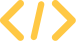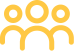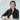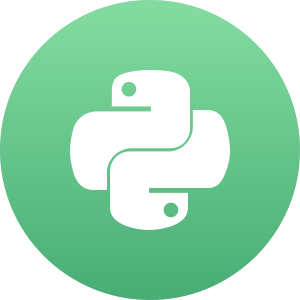# Do Left-handed People Really Die Young?

Use pandas and Bayesian statistics to see if left-handed people actually die earlier than righties.

Start Project
•10 tasks
•1,340 participants
•1,500 XP

#### Project Description

Barack Obama is left-handed. So are Bill Gates and Oprah Winfrey; so were Babe Ruth and Marie Curie. A 1991 study reported that left-handed people die on average nine years earlier than right-handed people. Nine years! Could this really be true?

In this project, you will explore this phenomenon using age distribution data to see if we can reproduce a difference in average age at death purely from the changing rates of left-handedness over time, refuting the claim of early death for left-handers. This notebook uses `pandas` and Bayesian statistics to analyze the probability of being a certain age at death given that you are reported as left-handed or right-handed.

This project lets you apply the skills from pandas Foundations, Statistical Thinking in Python, Introduction to Data Visualization with Python, and concepts from Foundations of Probability in R and Fundamentals of Bayesian Data Analysis in R, including reading data, creating new columns, creating matplotlib visualizations, using numpy arrays, constructing probabilities from frequency data, and Bayes' theorem. We recommend that you take those courses before starting this project.

• 1Where are the old left-handed people?
• 2Rates of left-handedness over time
• 3Applying Bayes' rule
• 4When do people normally die?
• 5The overall probability of left-handedness
• 6Putting it all together: dying while left-handed (i)
• 7Putting it all together: dying while left-handed (ii)
• 8Plotting the distributions of conditional probabilities
• 9Moment of truth: age of left and right-handers at death•Python